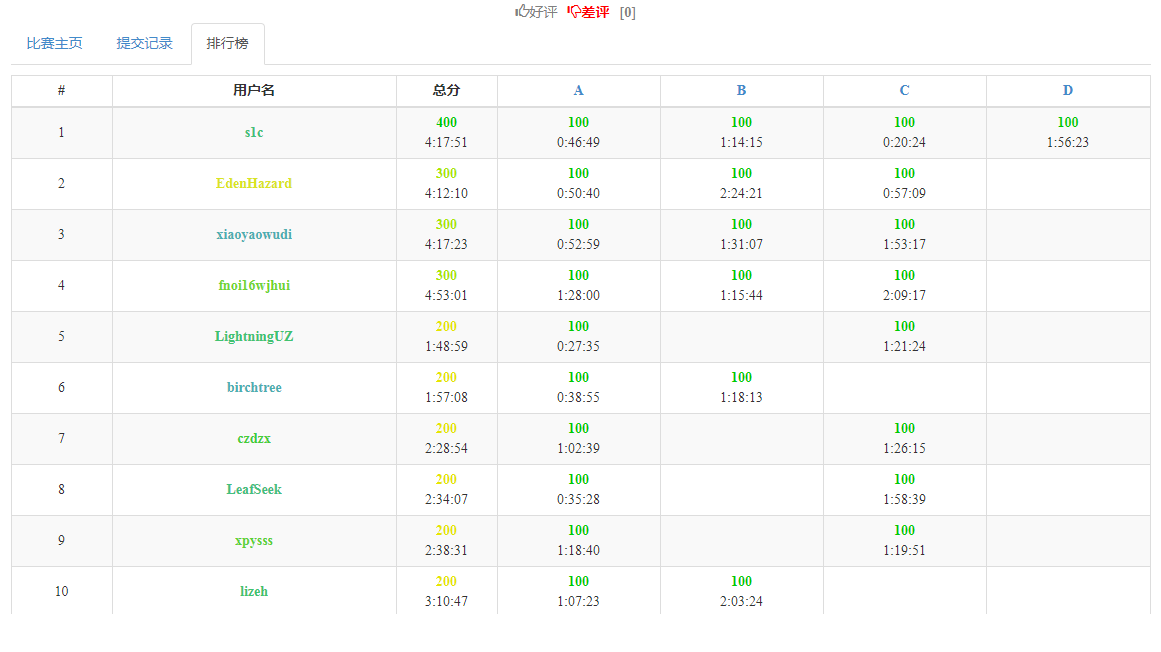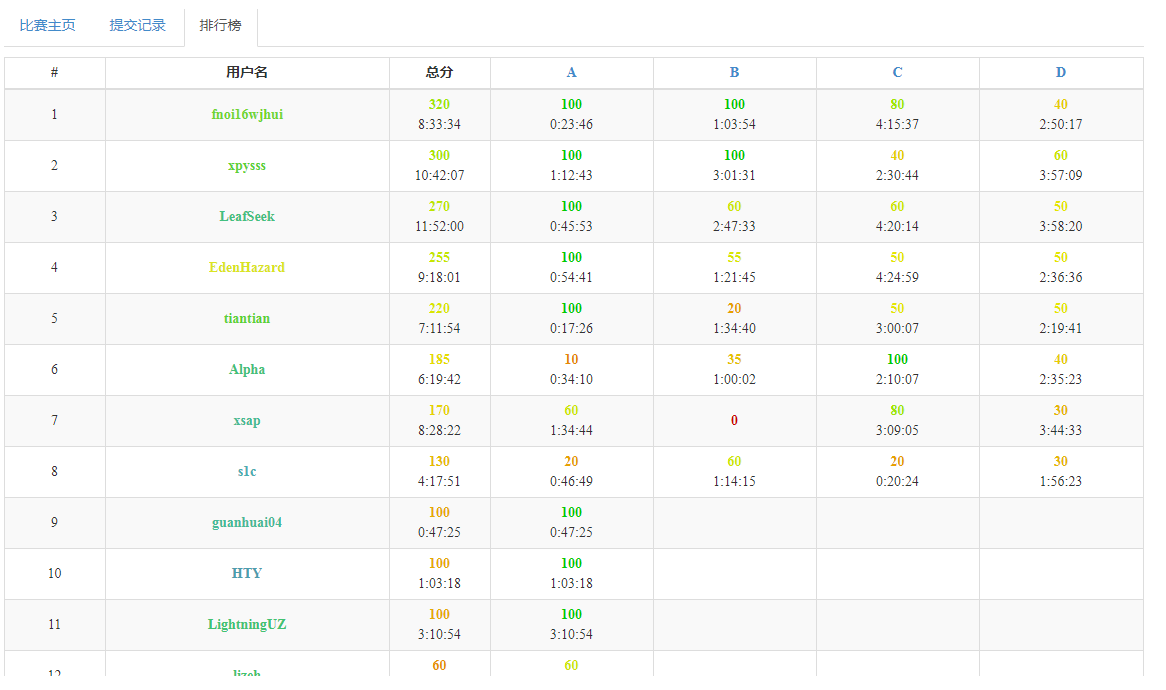# NOI.AC

## 新版马保国

2020-11-21 11:10:08 By s1c## 评论

FreeMiniWorld
$2^{2^{2^{2^{2^{2^{2^{2^{2^{2^{2^{2^{2^{2^{2^{2^{2^{2^{2^{2^{2^{2^{2^{2^{2^{2^{2^{2^{2^{2^{2^{2^{2^{2^{2^{2^{2^{2^{2^{2^{2^{2^{2^{2^{2^{2^{2^{2^{2^{2^{2^{2^{2^{2^{2^{2^{2^{2^{2^{2^{2^{2^{2^{2^{2^{2^{2^{2^{2^{2^{2^{2^{2^{2^{2^{2^{2^{2^{2^{2^{2^{2^{2^{2^{2^{2^{2^{2^{2^{2^{2^{2^{2^{2^{2^{2^{2^{2^{2^{2^{2^{2^{2^{2^{2^{2^{2^{2^{2^{2^{2^{2^{2^{2^{2^{2^{2^{2^{2^{2^{2^{2^{2^{2^{2^{2^2}}}}}}}}}}}}}}}}}}}}}}}}}}}}}}}}}}}}}}}}}}}}}}}}}}}}}}}}}}}}}}}}}}}}}}}}}}}}}}}}}}}}}}}}}}}}}}}}}}}}}}}}}}}}}}}}}}}}}}}}}}}}}$
ACwisher
$6^6^6^6^6^6^6^6^6^6^6^6^6^6^6^6^6^6^6^6^6^6^6^6^6^6^6^6^6^6^6^6^6^6^6^6^6^6^6^6^6^6^6^6^6^6^6^6^6^6^6^6^6^6^6^6^6^6^6^6^6^6^6^6^6^6^6^6^6^6^6^6^6^6^6^6^6^6^6^6^6^6^6^6^6^6^6^6^6^6^6^6^6^6^6^66^6^6^6^6^6^6^6^6^6^6^6^6^6^6^6^6^6^6^6^6^6^6^6^6^6^6^6^6^6^6^6^6^6^6^6^6^6^6^6^6^6^6^6^6^6^6^6^6^6^6^6^6^6^6^6^6^6^6^6^6^6^6^6^6^6^6^6^6^6^6^6^6^6^6^6^6^6^6^6^6^6^6^6^6^6^6^6^6^6^6^6^6^6^6^66^6^6^6^6^6^6^6^6^6^6^6^6^6^6^6^6^6^6^6^6^6^6^6^6^6^6^6^6^6^6^6^6^6^6^6^6^6^6^6^6^6^6^6^6^6^6^6^6^6^6^6^6^6^6^6^6^6^6^6^6^6^6^6^6^6^6^6^6^6^6^6^6^6^6^6^6^6^6^6^6^6^6^6^6^6^6^6^6^6^6^6^6^6^6^66^6^6^6^6^6^6^6^6^6^6^6^6^6^6^6^6^6^6^6^6^6^6^6^6^6^6^6^6^6^6^6^6^6^6^6^6^6^6^6^6^6^6^6^6^6^6^6^6^6^6^6^6^6^6^6^6^6^6^6^6^6^6^6^6^6^6^6^6^6^6^6^6^6^6^6^6^6^6^6^6^6^6^6^6^6^6^6^6^6^6^6^6^6^6^66^6^6^6^6^6^6^6^6^6^6^6^6^6^6^6^6^6^6^6^6^6^6^6^6^6^6^6^6^6^6^6^6^6^6^6^6^6^6$
ios
orz
fake_H6_6Q
$2^{2^{2^{2^{2^{2^{2^{2^{2^{2^{2^{2^{2^{2^{2^{2^{2^{2^{2^{2^{2^{2^{2^{2^{2^{2^{2^{2^{2^{2^{2^{2^{2^{2^{2^{2^{2^{2^{2^{2^{2^{2^{2^{2^{2^{2^{2^{2^{2^{2^{2^{2^{2^{2^{2^{2^{2^{2^{2^{2^{2^{2^{2^{2^{2^{2^{2^{2^{2^{2^{2^{2^{2^{2^{2^{2^{2^{2^{2^{2^{2^{2^{2^{2^{2^{2^{2^{2^{2^{2^{2^{2^{2^{2^{2^{2^{2^{2^{2^{2^{2^{2^{2^{2^{2^{2^{2^{2^{2^{2^{2^{2^{2^{2^{2^{2^{2^{2^{2^{2^{2^{2^{2^{2^{2^{2^2}}}}}}}}}}}}}}}}}}}}}}}}}}}}}}}}}}}}}}}}}}}}}}}}}}}}}}}}}}}}}}}}}}}}}}}}}}}}}}}}}}}}}}}}}}}}}}}}}}}}}}}}}}}}}}}}}}}}}}}}}}}}}$
cqh2025
$2^{2^{2^{2^{2^{2^{2^{2^{2^{2^{2^{2^{2^{2^{2^{2^{2^{2^{2^{2^{2^{2^{2^{2^{2^{2^{2^{2^{2^{2^{2^{2^{2^{2^{2^{2^{2^{2^{2^{2^{2^{2^{2^{2^{2^{2^{2^{2^{2^{2^{2^{2^{2^{2^{2^{2^{2^{2^{2^{2^{2^{2^{2^{2^{2^{2^{2^{2^{2^{2^{2^{2^{2^{2^{2^{2^{2^{2^{2^{2^{2^{2^{2^{2^{2^{2^{2^{2^{2^{2^{2^{2^{2^{2^{2^{2^{2^{2^{2^{2^{2^{2^{2^{2^{2^{2^{2^{2^{2^{2^{2^{2^{2^{2^{2^{2^{2^{2^{2^{2^{2^{2^{2^{2^{2^{2^2}}}}}}}}}}}}}}}}}}}}}}}}}}}}}}}}}}}}}}}}}}}}}}}}}}}}}}}}}}}}}}}}}}}}}}}}}}}}}}}}}}}}}}}}}}}}}}}}}}}}}}}}}}}}}}}}}}}}}}}}}}}}}$
kkksb03

kkksb03